# 15.10 - Using Extrapolation to Replace Stale Statistics - Teradata Database

## Teradata Database SQL Request and Transaction Processing

prodname
vrm_release
15.10
category
Programming Reference
User Guide
featnum
B035-1142-151K

Users often query their databases over date intervals for which one or both bounds on a predicate are dates for rows that have been inserted into a table since the last time statistics were collected for the date columns specified for that table in the predicate.

Extrapolating statistics enables you to submit, for example, a query specifying a date range predicate in which one or all of the specified dates is past the latest date stored in the statistical histograms for the DATE column set. To support such queries, the Optimizer applies an extrapolation technique to make a reasonable estimate for the rows that have been inserted after statistics were last collected without requiring them to be recollected. Extrapolation methods for statistics can be viewed as a form of derived statistics (see “Derived Statistics” on page 210).

In this context, the definition of a future date applies only to dates occurring between the time statistics were last collected on a DATE column and the current date. In other words, future is a narrowly defined, relative term, and extrapolation does not apply to data having true future dates that cannot exist at the time you submit your date‑extrapolation‑requiring queries. Rather, the term applies to dates that are otherwise in a statistical limbo.

The optimizer attempts to extrapolate the following demographics.

• Table cardinality
• Teradata Database uses extrapolation from the following information to update table cardinalities.

• Histogram summary information
• System UDI counts if they are available, reliable, and not obsolete
• History data
• Single‑AMP or all‑AMP sampling
• Stale histograms, both for the number of distinct values and for the maximum value of the histogram
• The extrapolation methods used depend on whether Teradata Database determines that a column is categorized as rolling, static, or a hybrid (see “Definitions of Rolling, Static, and Hybrid Columns” on page 293).

Extrapolation logic estimates the data growth from the last time statistics were collected or refreshed in order to detect stale statistics and apply extrapolations. The Optimizer combines cardinality estimates derived from both UDI counts and extrapolation logic to make accurate cardinality estimates, and making stale statistics detection more robust. UDI counts also enable the Optimizer to perform interpolations because of the improved accuracy of data changes provided by the tracking of UDI counts.

Teradata Database also uses extrapolation to estimate cardinalities based on dynamic single‑ or all‑AMP samples (see “Using Extrapolated Cardinality Estimates to Assess Table Growth” on page 290).

The ability of the Optimizer to extrapolate statistics does not remove the need to recollect statistics.

The relative accuracy of residual (residual meaning existing statistics that were not collected recently) statistics for static columns (see “Definitions of Rolling, Static, and Hybrid Columns” on page 293) with respect to a dynamic AMP sample (in this section, the term dynamic AMP sample refers to dynamic single AMP sampling only.), and whether residual statistics should be considered to be stale, depends on several factors. Residual statistics are not necessarily stale statistics.

The term residual statistics implies the likelihood that those statistics no longer provide an accurate picture of current column data. This is not necessarily a correct assumption.

You can protect yourself from recollecting statistics unnecessarily by specifying various threshold options when you submit your initial COLLECT STATISTICS requests on an index or column set. See “COLLECT STATISTICS (Optimizer Form” in SQL Data Definition Language for more information about using threshold options to recollect statistics.

If the relative demographics for a column set do not change, then residual statistics are normally a reliable representation of the current demographics of an index or column set. The comparison made by the derived statistics framework between residual statistics, if they exist, and a dynamic AMP sample makes the final determination of whether the Optimizer considers the residual statistics to be stale or not.

It is possible for the cardinality of a table to grow by more than 10%, but for the relative proportion of particular values in its rows not to change. This so-called Ten Percent Rule also applies at the partitioning level for row‑partitioned tables. If the number of changed row partitions exceeds 10% of the total number of row partitions for the table (in other words, if more than 10% of the rows are added to or deleted from a row partition), then you should recollect statistics on the index. For row‑partitioned tables and column‑partitioned tables, any refreshment of statistics should include the system‑derived PARTITION column.

As a result of these safeguards, even a change in the table demographics of this magnitude might not affect the query plan generated by the Optimizer.

When this is true, residual statistics can still be more accurate than a newly collected dynamic AMP sample, though even a single‑AMP dynamic AMP sample, depending to some degree on whether the column being sampled is indexed or not, typically provides excellent cardinality estimates (see, for example, “Dynamic AMP Sampling of NUSIs” on page 184). The accuracy of the statistics collected from a dynamic AMP sample also depends to a relatively small degree on the number of AMPs sampled, which is determined by the setting of an internal DBS Control field. The possibilities range through 1, 2, or 5 AMPs, all AMPs on a node, or all AMPs on a system. Consult your Teradata support representative for details.

The Optimizer uses SUMMARY statistics to establish an estimate of the base cardinality of a table. Teradata Database then extrapolate the cardinality by comparing the saved dynamic sample from the SUMMARY statistics with the fresh sample. The system takes the difference between the new and old samples as the growth in cardinality of the table.

The Optimizer estimates table cardinalities from SUMMARY statistics, which you can collect or recollect explicitly by submitting an appropriate COLLECT SUMMARY STATISTICS request or by collecting or recollecting statistics on any column set that indirectly updates the SUMMARY statistics, and dynamic AMP samples or collected PARTITION statistics, which can be gathered for all tables, whether they are partitioned or not. The collection of SUMMARY statistics takes advantage of several optimizations that make the process run very quickly. The ideal choice, then, is to provide accurate cardinality estimates to the Optimizer using the method with the least overhead.

Teradata Database compares the fresh dynamic AMP sample fetched at the time a request is compiled with the sample from the SUMMARY statistics, and the change in growth of the table is determined from that comparison. Even if the absolute estimate of the sample is not exact, the rate of growth is accurate as long as it is uniform across all of the AMPs on the system because the same AMP is used to collect a “dynamic” statistical sample each time a request is compiled (see “Dynamic AMP Sampling” on page 181 for details).

At a very high level, the overall process is as follows.

1 Retrieve the cardinality estimates for the table from the table descriptor and from a dynamic AMP sample.

2 Determine whether SUMMARY statistics are available for the table.

If so, use them to estimate its cardinality.

3 If SUMMARY statistics are not available, use a dynamic AMP sample to estimate the cardinality of the table.

 IF the sample is taken from … THEN set its confidence to … a single AMP Low all AMPs High

See “About Optimizer Confidence Levels” on page 537 for descriptions of the various Optimizer confidence levels.

4 If the relation is a compressed join index, estimate the cardinality to be equal to the cardinality estimated from its SUMMARY statistics.

Otherwise, extrapolate the estimated cardinality as the cardinality estimated from the SUMMARY statistics.

Consider a more detailed scenario. Suppose the Optimizer evaluates statistics to determine if NUSIs are to be used in the query plan. The standard evaluation criterion for determining the usefulness of a NUSI in a query plan is that the number of rows that qualify per data block should be less than 1.

Assume an average data block size of 50 KB and an average row size of 50 bytes. This produces an average of 1,000 rows per data block. Suppose the number of rows for a particular NUSI is 1 in every 2,000 rows, or one row in every 2 data blocks. The Optimizer determines that using the NUSI will save reading some significant number of data blocks, so employing it in the query plan would result in fewer I/Os than doing a full‑table scan.

Now, assume the table grows by 10%. The number of qualifying rows is now 1 in every 2,200 rows (a 10% increase in the cardinality of the table). For this particular case, the number of rows per data block is still less than 1, so the Optimizer does not need new statistics to produce a good query plan, and the derived statistics framework detects this.

On the other hand, consider a join scenario in which the Optimizer needs to estimate how many rows will qualify for spooling. This can be critical, especially if the original estimate is near the cusp of the crossover point where a 10% increase in the number of rows makes the Optimizer change its selection from one join plan to another.

Without the derived statistics framework being able to detect whether the residual statistics are stale or not, working with them could have meant that the Optimizer would have chosen a bad query plan instead of a new, faster plan. Or, worse still, the residual statistics could produce a new join plan that is much slower than the previous plan.

The Optimizer attempts to extrapolate the following demographics.

• Table cardinality
• Stale statistics
• When possible, the Optimizer uses extrapolation to derive estimates of the following statistics when they are determined to be stale.

• Number of distinct values
• Interval maximum values
• Note that the Optimizer can also use interpolation of demographics to estimate table demographics.

When data distribution is relatively uniform within the collected set of statistics, extrapolation can provide a reasonably accurate estimate of future cardinalities. However, if there are many spikes in the existing statistics, or if they are overly skewed, then you should recollect statistics on the column or index because extrapolation can produce inaccurate estimates in such situations and cannot be relied upon to produce accurate cardinality estimates.

The Optimizer can only apply extrapolation for statistics on a single column or single‑column index on a column. The performance impact for applying extrapolation in a request is minimal.

The Optimizer performs the following extrapolations in the order indicated when it determines that the statistics it needs are stale.

1 Extrapolation of table cardinalities.

2 Extrapolation of distinct values for rolling columns.

3 Extrapolation of cardinalities for rolling and static columns.

See “Definitions of Rolling, Static, and Hybrid Columns” on page 293 for definitions of these terms.

Note that the system only extrapolates row and distinct value cardinalities upward.

The Optimizer depends on UDI counts, table‑level statistical information, and table‑level history to estimate table cardinality accurately.

The process begins by retrieving the row count from the table‑level demographics and treats it as a base row count of the table.

The Optimizer uses information like UDI counts, dynamic AMP samples, and table‑level history data to validate the adjusted cardinality. If the adjusted row count is significantly different than the UDI counts, dynamic AMP sample, or table‑level history suggest, the Optimizer readjusts the row count.

The most obvious application of extrapolated statistics, deriving extrapolated date column statistics to better optimize range queries over a future date or dates (see, for example, “Closed Range Date Predicate: Case 2” on page 299), improves the accuracy of the cardinality estimates the Optimizer uses to create the portion of the query plan for a request that applies to the given date predicate. For this application, the term future means any rows inserted into a table between the date statistics were last collected on the relevant date column or index and its extrapolated boundary date.

Extrapolating statistics does not do any of the following things.

• Change the procedure for collecting or dropping statistics
• Store the extrapolated statistics in the relevant interval histogram
• Affect the type of information reported by a HELP STATISTICS request
• Note that date extrapolation does affect the specific cardinality information reported for a query EXPLAIN report, not by adding new terminology, but because of the different, and more accurate, cardinality estimates it produces.

The Optimizer considers extrapolating date statistics only when it detects that the existing statistics on the relevant date column or index are stale. In this particular case, the term stale applies to statistics that predate any of the values specified in a predicate that ranges over date values.

The following terminology is used for the examples of extrapolated date statistics.

 Term Equation  Variable Definition Extrapolated boundary `  e` The upper boundary for extrapolated statistics for future date values. Lower boundary on collected statistics `  l` The oldest date for which statistics have been collected on date values of a column or index. Upper boundary on collected statistics `  h` The most recent date for which statistics have been collected on date values of a column or index. Number of unique values `  v` The number of unique values among the collected statistics value set. Extrapolated number of unique values `  v’` The additional number of unique values in the extrapolated statistics as estimated from a dynamic AMP sample.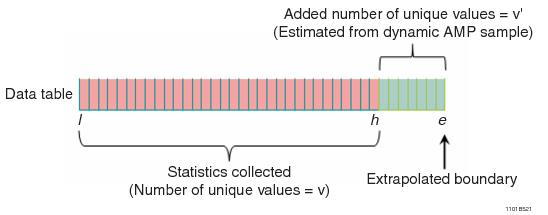The Optimizer extrapolates or interpolates cardinalities using different methods depending on whether the values being extrapolated are from a rolling column, a static column, or a hybrid column. The following table defines these terms.

 Term Definition Rolling column A column with a constant number of rows per value such as dates and timestamps. For these columns, the demographics of existing data never change and only new data gets new distinct values. Columns having a data type of DATE or TIMESTAMP and columns that are unique or 95% or more unique are classified as rolling columns. For example, suppose you have a column order_date in an orders table. The demographics of the old order dates never change, and only new orders are added to the table. Static column A column with varying rows per value such as product_id, business_unit_id, dept_id, and the like, and a constant number of unique values. The Optimizer assumes that few new values are inserted into a static column across time. For this type of column, newly added data can change the demographics of the historical or existing data. For example, orders that are newly added to an orders table can change the number of orders for a given product ID. When a static column has stale statistics, the Optimizer can make a good extrapolated estimate if the system can estimate the table cardinality growth accurately. Hybrid column A column with both rolling unique values and varying rows per value. For hybrid columns, newly added data changes the rows per value of the old data values and also adds new data values. Examples of hybrid column are columns like product_code and supplier_code. The new data includes the sales for the existing products and at the same time sales of the newly added products.

The Optimizer uses data from the history intervals of an interval histogram to detect the data pattern for a given column. Once it determines the historical pattern of the column, it assigns that column to one of the previously listed categories. If no history intervals are available for a column, the Optimizer classifies it as either a rolling column or a static column using whatever data is available to make that determination.

Extrapolation thresholds are required to guard against inaccuracies in dynamic‑AMP samples.

Note that all‑AMP sampling overrides the default thresholds.

For rolling columns, the Optimizer extrapolates the maximum value based on the newly added data and then bases its selectivity estimates on the newly determined maximum. The new maximum is determined from the data growth and the rows per value as described below.

• New number of distinct values = newly added number of rows per average rows per value.
• New maximum = old maximum from the stale histogram + new number of distinct values.
• The following graphic illustrates examples of extrapolation for rolling columns.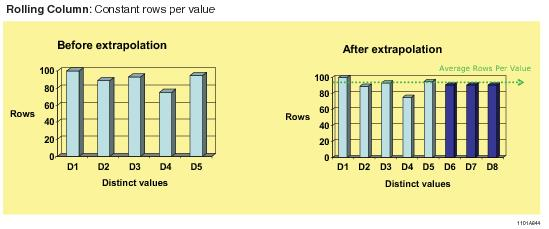Consider the following simple example.

Assume you have an orders table with an average of 1 million orders per day and you collect statistics on the order_date column on July 25, 2010. If the Optimizer detects a growth of 2 million, it extends the histogram maximum date to Jul 27, 2010, assuming an average of 1 million rows per day.

For a condition such as `order_date BETWEEN '2010-26-07' AND '2010-27-07'`, the Optimizer estimates the number of qualified rows to be 2 million.

If extrapolation logic were not in place to deduce the maximum value, the estimate of the number of qualified rows returned by the Optimizer would be 0 rows.

Note that the maximum value extrapolation logic has no bearing on the current date. Teradata Database extends the previous maximum from the stale histogram to estimate the new maximum purely based on the data growth and the average rows per value.

In addition to the row count extrapolation, the Optimizer also extrapolates the number of distinct values. For the preceding example, the Optimizer adds 2 distinct values, for 2 additional days’ worth of data, to the existing value count for order_date.

The extrapolation logic for rolling columns has the following properties.

• Acts only when the Optimizer detects a stale histogram whose statistics are needed for a request.
• Calculates an upper boundary to limit the extrapolation to values it can estimate with confidence (see “Extrapolated Boundaries for Date Statistics” on page 292).
• Estimates and then adds “missing” rows for both closed and open range intervals (see “Closed Range Date Predicate: Case 1” on page 298 - “Simple Open Range Predicate” on page 301.
• Extrapolates values for columns with very large highest date values, which translates to a wide final interval in the histogram (see “Open Range Predicate With Wide Final Interval” on page 298, for example).
• For example, if the interval histogram indicates the table cardinality as 500 rows with 250 unique values, but the table cardinality is actually 1,000 rows, Teradata Database scales the number of unique values up to 500 by assuming that the constant number of rows per unique value is 2, so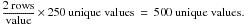Furthermore, for any rolling column defined to be UNIQUE, the Optimizer scales the extrapolated number of distinct values upward to equal the table cardinality by default.

If the Optimizer detects stale statistics for a rolling column, it extrapolates the number of distinct values assuming that the number of rows per value is constant. The following flowchart provides a rough approximation of the algorithm used to estimate the extrapolated cardinalities of rolling and static columns.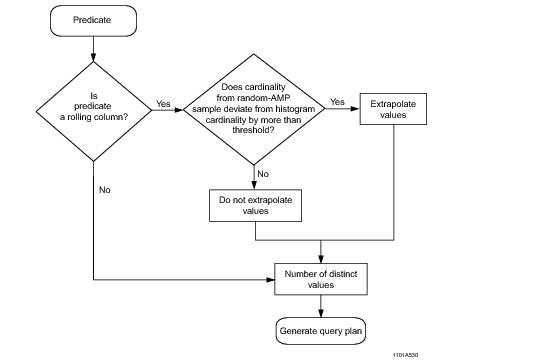The Optimizer extrapolates the maximum value for rolling columns based on newly added data. The Optimizer then makes its selectivity estimates using the new maximum. Teradata Database determines the new maximum based on the amount of data growth and the rows per value as described by the following bullets.

• New number of distinct values = newly added number of rows per average rows per value
• New maximum = old maximum from the stale histogram + new number of distinct values
• Consider the following example. Suppose that you have an orders table with an average of 1 million orders per day, and you collect statistics on the order_date column on July 25, 2010. If the Optimizer detects growth of 2 million rows, it extends the histogram maximum date to July 27, 2010, assuming an average of 1 million rows per day.

For a condition such as `order_date BETWEEN '2010-26-07' AND '2010-27-07'`, the Optimizer estimates the number of qualified rows to be 2 million.

If extrapolation logic were not in place to deduce the maximum value, the estimate returned by the Optimizer would have been 0 rows.

Note that the maximum value extrapolation logic has no bearing on the current date. The Optimizer extends the previous maximum from the stale histogram to get the new maximum based only on the data growth and the average rows per value.

In addition to the row count extrapolation, the Optimizer also extrapolates the number of distinct values. For this example, it makes the estimate of the number of distinct values by adding 2 distinct values (for 2 more days worth of data) to the existing value count of order_date.

For static columns with stale statistics, the Optimizer can use extrapolation to replace those statistics with an extrapolated estimate provided that the added cardinality resulting from table growth can be estimated accurately.

The following graphic illustrates examples of extrapolation for static columns.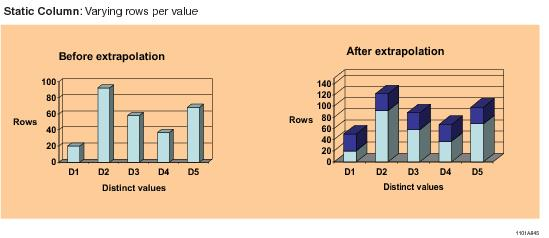For static columns, the Optimizer distributes the data growth that it detects evenly among all the distinct values to estimate the extrapolated row counts per value.

For example, if there are 100 products in the orders table and the growth is 2 million rows, the Optimizer divides the 2 million rows evenly among these 100 products to make its extrapolated estimate. For a request that has the predicate `product_cd=5`, if the old frequency of product code 5 is 50,000 rows, the Optimizer estimates the new frequency to be 50,000 + 20,000 rows based on the extrapolated growth. The value 20,000 is based on the extrapolated growth for product code 5. The final estimate for the predicate is 70,000 rows.

The number of distinct values remains the same for static columns.

For hybrid columns, the Optimizer uses histogram history data to estimate the number of new distinct values and the strategies to distribute the newly added data to the existing values.

The Optimizer assumes the number of rows per value for newly added data from the current histogram statistics. The system mines the history data to get a picture of how many new values have been added for a given amount of data.

Using the rows per value and the estimated number of distinct values, Teradata Database divides the new data into 2 buckets.

• Data that belongs to the newly added distinct values.
• Teradata Database distributes the data using the number of rows per value for the newly added values.

• Data that belongs to the existing distinct values.
• Data is distributed among the existing distinct values based on the approach described previously for static columns.

Stale statistics on views are also extrapolated using the same techniques once the history is established that reveals the growth of these statistics.

Consider the following request over the open range bounded by May 30, 2007 on the lower end and unbounded on the upper end.

`     SELECT *`
`     FROM ordertbl`
`     WHERE o_orderdate >= DATE ‘05-30-2007’;`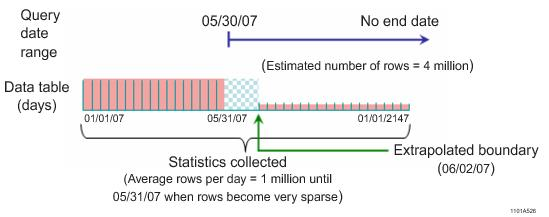Unlike the previous cases, the average number of rows inserted into ordertbl is a constant one million per day until May 31, 2007, at which point the number of new rows inserted into the table becomes very sparse. Because the dates for the projected values extend so far into the future, the last interval in the equal‑height histogram is extremely wide.

A dynamic AMP sample provides the Optimizer with enough information to detect the change in the rate of row insertion and to compensate accordingly in calculating an extrapolated date for the request, which is June 6, 2007, only 8 days past the date the rate of row insertion changed radically. As a result, the Optimizer uses an approximate extrapolated cardinality of 8 million rows when it develops its query plan, which is 4 times larger than the number that would be estimated without extrapolation.

Consider the following request over the closed range bounded on the lower end by July 17, 2007 and on the upper end by July 23, 2007.

`     SELECT *`
`     FROM ordertbl`
`     WHERE o_orderdate BETWEEN DATE ‘07-17-2007’`
`                       AND     DATE ‘07-23-2007’;`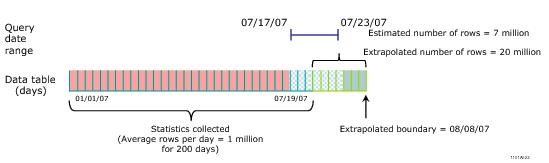Statistics have been collected on o_orderdate over the date range of January 1, 2007 through July 19, 2007, inclusive.

Substituting the dates for the existing upper and lower bounds on collected statistics, the known number of unique values in those statistics, and the dynamic AMP sample‑based estimate of the number of additional unique values into the equation for determining a date extrapolation boundary, you obtain the following result.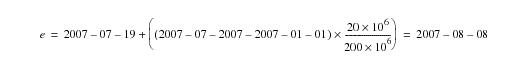So based on the assumptions made for calculating an extrapolated upper boundary, it is determined that the extrapolated boundary for the new statistics is August 8 2007, and the Optimizer estimates an approximate cardinality of 7 million rows for the result, which is more than twice the cardinality that would be estimated without extrapolation, which is 3 million rows.

The closed range predicate in the request is covered within this extrapolated boundary,

Consider the following request over the closed range bounded on the lower end by August 6, 2007 and on the upper end by August 11, 2007.

`     SELECT *`
`     FROM ordertbl`
`     WHERE o_orderdate BETWEEN DATE ‘08-06-2007’`
`                       AND     DATE ‘08-11-2007’;`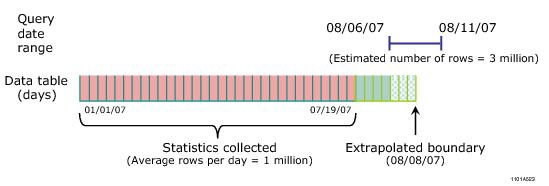Statistics have been collected on o_orderdate over the date range of January 1, 2007 through July 19, 2007, inclusive.

Substituting the dates for the existing upper and lower bounds on collected statistics, the known number of unique values in those statistics, and the dynamic AMP sample‑based estimate of the number of additional unique values into the equation for determining a date extrapolation boundary, the extrapolated boundary for the new statistics is determined to be August 8 2007, just as in “Closed Range Date Predicate: Case 1” on page 298.

Unlike the situation for Case 1, however, the upper bound on the closed range of the predicate falls outside the extrapolated boundary date for the statistics. The Optimizer is still able to estimate an approximate cardinality of 3 million rows for the result, which is 6 orders of magnitude better than the estimate that would be made without extrapolating statistics to the August 8, 2007 date, which would have been 1 row.

Consider the following request over the open range bounded on the lower end by July 16, 2007 and unbounded on the upper end.

`     SELECT *`
`     FROM ordertbl`
`     WHERE o_orderdate >= DATE ‘07-16-2007’;`

Statistics have been collected on o_orderdate over the date range of January 1, 2007 through July 19, 2007, inclusive.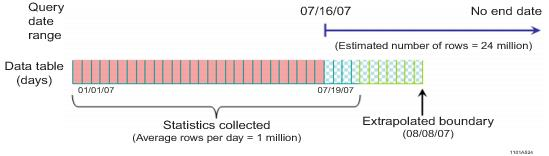Substituting the known data into the equation for determining a date extrapolation boundary returns August 8, 2007, as it does for all these date range predicate examples because they are all calculated using the same base of collected statistics and average number of rows inserted per day over the interval on which those statistics were collected.

Unlike the previous cases, the upper bound on the range of the predicate is open, so it falls outside the extrapolated boundary date for the statistics by definition. The Optimizer still is able to estimate an approximate cardinality of 24 million rows for the result, which is nearly an order of magnitude better than the estimate that would be made without extrapolating statistics to the August 8, 2007 date, which is 4 million rows.

Consider the following request over the open range bounded on the lower end by September 1, 2007 and unbounded on the upper end.

`     SELECT *`
`     FROM ordertbl`
`     WHERE o_orderdate >= DATE ‘08-04-2007’;`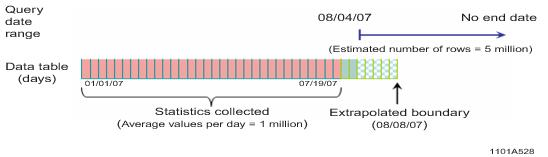The lower boundary on this interval is within the extrapolated boundary for the statistics on the o_orderdate column, so the Optimizer is able to estimate a cardinality for the result, which is approximately 5 million rows. This result is 6 orders of magnitude better than the estimate that would be made without extrapolating the statistics to the August 8, 2007 date, which is
1 row.

Consider the following request over the open range bounded by September 1, 2007 on the lower end and unbounded on the upper end.

`     SELECT *`
`     FROM ordertbl`
`     WHERE o_orderdate >= DATE ‘09-01-2007’;`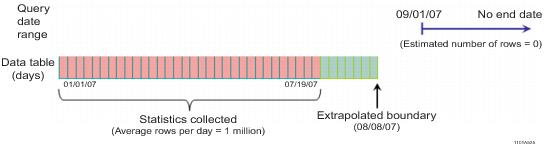Because the lower boundary on this interval is past the extrapolated boundary for the statistics on the o_orderdate column, the Optimizer cannot estimate an approximate cardinality for the result, so it projects a result of 0 rows with or without using extrapolation.

Consider the following request specifies an IN list predicate with widely separated values that are specified over the static column ps_suppkey.

`     SELECT *`
`     FROM partsupp`
`     WHERE ps_suppkey IN (4, 35000, 40000);`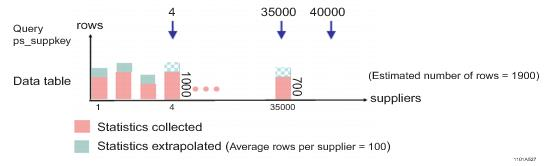Because ps_suppkey is a static column, the Optimizer assumes that virtually no new ps_suppkey values will be added to the partsupp table in the future, so it uses a simple dynamic‑AMP sample to determine the extrapolated number of new rows having the values 4, 35000, or 40000 for ps_suppkey.

Assuming that the number of rows per value is a constant sum across all values of partsupp, but that all or some of the needed values are not in the range of the values contained in the collected statistics, the system extrapolates a solution using one of three methods.

 IF the … THEN the number of rows per value is taken from … value is less than the MinValue in the interval the first interval in the histogram. value is greater than the MaxValue in the interval the last interval in the histogram. first or last intervals are either high‑biased or single‑value equal‑height intervals the table‑level estimated rows per value based on a dynamic AMP sample.

The estimated cardinality is then accumulated for each element of the IN list or OR list predicate to determine the final estimate. The maximum number of elements you can specify in an IN list or OR list is 1,048,576.

In this particular case, there is no ps_suppkey value of 40,000, and because ps_suppkey is a static column, the Optimizer assumes that the value 40,000 is not likely to have been added since statistics were last collected. It uses a dynamic‑AMP sample to extrapolate an estimate of 100 additional rows per ps_suppkey value based on the existing average rows inserted daily per value.

The final approximate cardinality estimates are 1,000+100=1,100 rows for ps_suppkey=4 and 700+100=800 rows for ps_suppkey=35,000. Without extrapolation, the Optimizer estimates cardinalities of 1,000 rows for ps_suppkey=4 and 700 rows for ps_suppkey=35,000, both of which are taken directly from the existing interval histogram statistics (see “Definitions of Rolling, Static, and Hybrid Columns” on page 293).